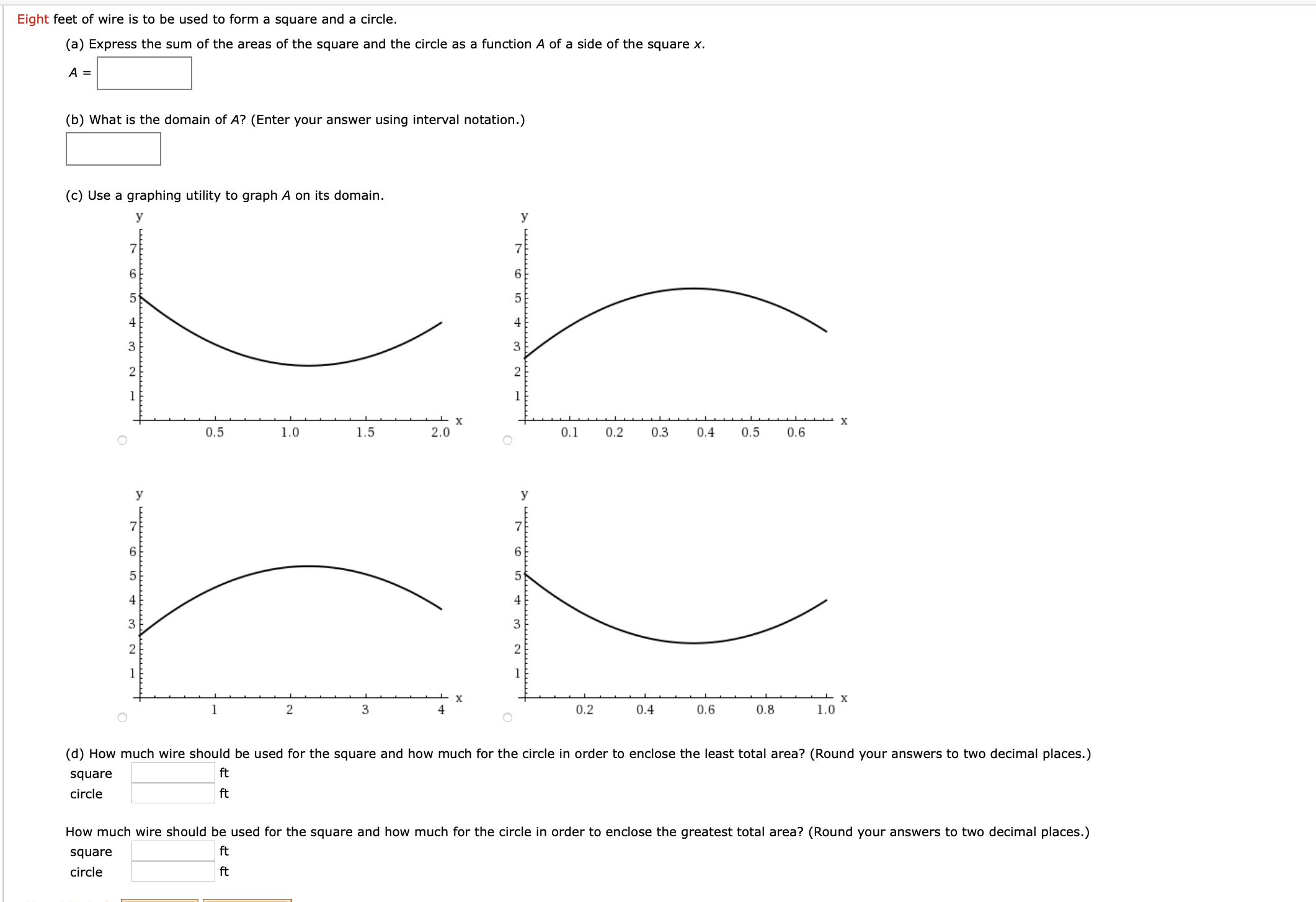# Eight feet of wire is to be used to form a square and a circle. (a) Express the sum of the areas of the square and the circle as a function A of a side of the square x. A = (b) What is the domain of A? (Enter your answer using interval notation.) (c) Use a graphing utility to graph A on its domain. y У 6. 4 4 3 х х 0.5 1.0 1.5 2.0 0.1 0.2 0.3 0.4 0.5 0.6 У 6. 4 4 3 3 2 3 4 0.2 0.4 0.6 0.8 1.0 (d) How much wire should be used for the square and how much for the circle in order to enclose the least total area? (Round your answers to two decimal places.) square ft circle ft How much wire should be used for the square and how much for the circle in order to enclose the greatest total area? (Round your answers to two decimal places.) square ft circle ft

Question

How do you answer the question in the attached picture?help_outlineImage TranscriptioncloseEight feet of wire is to be used to form a square and a circle. (a) Express the sum of the areas of the square and the circle as a function A of a side of the square x. A = (b) What is the domain of A? (Enter your answer using interval notation.) (c) Use a graphing utility to graph A on its domain. y У 6. 4 4 3 х х 0.5 1.0 1.5 2.0 0.1 0.2 0.3 0.4 0.5 0.6 У 6. 4 4 3 3 2 3 4 0.2 0.4 0.6 0.8 1.0 (d) How much wire should be used for the square and how much for the circle in order to enclose the least total area? (Round your answers to two decimal places.) square ft circle ft How much wire should be used for the square and how much for the circle in order to enclose the greatest total area? (Round your answers to two decimal places.) square ft circle ft fullscreen